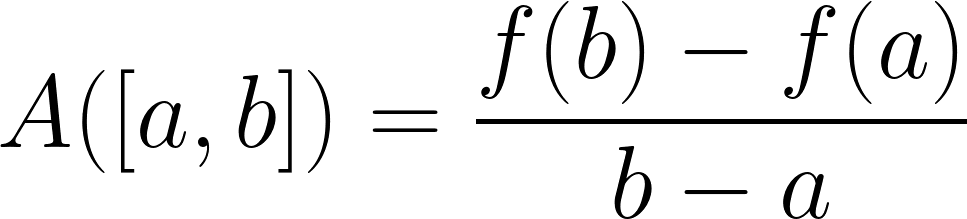# Average Rate of Change Calculator

Find the average rate of change by entering a function f(x) and an interval [a, b] in the calculator below.

## Average Rate of Change:

8

### Steps to Solve the Average Rate of Change

f(3) = 12

f(5) = 28

#### Step Two: Use the Average Rate of Change Formula

f(b) - f(a) / b - a

28 - 12 / 5 - 3

16 / 2

8

The average rate of change is equal to 8

Learn how we calculated this below

## How to Find the Average Rate of Change

The average rate of change is an average measure of change in a function over an interval. It’s the total change of the output of the function divided by the change in the function’s input.

To find the rate of change, first define the function that you’ll use to calculate it, along with an interval [a, b].

Then, you can find the average rate of change using the slope formula. The average rate of change is actually a slope, but rather than a linear slope where the average between any two points is equal to some constant, a function is used.

### Average Rate of Change Formula

Thus, the formula to find the average rate of change that is derived from the slope formula is:

average rate of change = Δy / Δx = y2 – y1 / x2 – x1 = f(b) – f(a) / b – a

The formula states that the average rate of change is equal to the result of the function of b f(b) minus the result of the function of a f(a), divided by the value of b minus the value of a.For example, let’s find the average rate of change for the interval [2, 3] using the function f(x) = x².

Start by replacing the values in the average rate of change formula.

average rate of change = 3² – 2² / 3 – 2

Then simplify.

average rate of change = 9 – 4 / 3 – 2
average rate of change = 5 / 1
average rate of change = 5

So, in this example, the average rate of change over the interval [2, 3] is equal to 5.